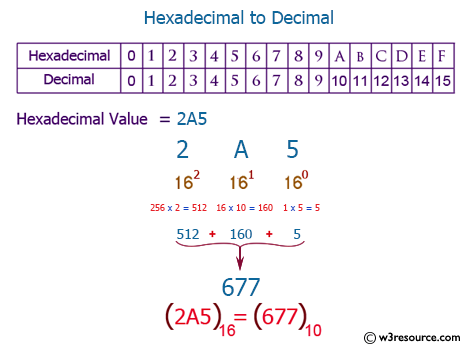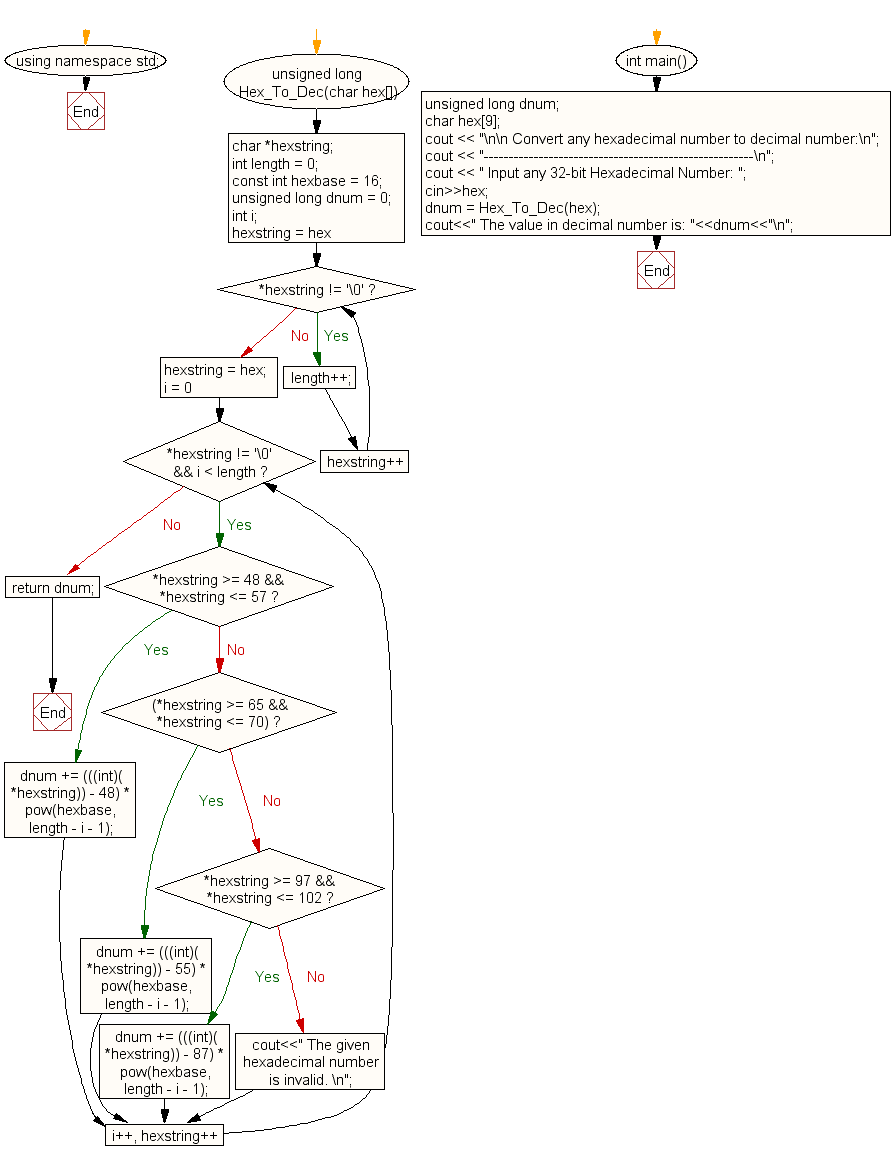﻿ C++ Exercises: Convert a hexadecimal number to decimal number - w3resource# C++ Exercises: Convert a hexadecimal number to decimal number

## C++ For Loop: Exercise-79 with Solution

Write a program in C++ to convert a hexadecimal number to decimal number.

Pictorial Presentation:Sample Solution:-

C++ Code :

``````#include<iostream>
#include<stdlib.h>
#include<math.h>
using namespace std;

unsigned long Hex_To_Dec(char hex[])
{
char *hexstring;
int length = 0;
const int hexbase = 16;
unsigned long dnum = 0;
int i;
for (hexstring = hex; *hexstring != '\0'; hexstring++)
{
length++;
}
hexstring = hex;
for (i = 0; *hexstring != '\0' && i < length; i++, hexstring++)
{
if (*hexstring >= 48 && *hexstring <= 57)
{
dnum += (((int)(*hexstring)) - 48) * pow(hexbase, length - i - 1);
}
else if ((*hexstring >= 65 && *hexstring <= 70))
{
dnum += (((int)(*hexstring)) - 55) * pow(hexbase, length - i - 1);
}
else if (*hexstring >= 97 && *hexstring <= 102)
{
dnum += (((int)(*hexstring)) - 87) * pow(hexbase, length - i - 1);
}
else {
cout<<" The given hexadecimal number is invalid. \n";
}
}
return dnum;
}
int  main()
{
unsigned long dnum;
char hex;
cout << "\n\n Convert any hexadecimal number to decimal number:\n";
cout << "------------------------------------------------------\n";
cout << " Input any 32-bit Hexadecimal Number: ";
cin>>hex;
dnum = Hex_To_Dec(hex);
cout<<" The value in decimal number is: "<<dnum<<"\n";
}
``````

Sample Output:

``` Convert any hexadecimal number to decimal number:
------------------------------------------------------
Input any 32-bit Hexadecimal Number: 25
The value in decimal number is: 37
```

Flowchart:C++ Code Editor:

Contribute your code and comments through Disqus.

What is the difficulty level of this exercise?

﻿

## C++ Programming: Tips of the Day

What is a smart pointer and when should I use one?

This answer is rather old, and so describes what was 'good' at the time, which was smart pointers provided by the Boost library. Since C++11, the standard library has provided sufficient smart pointers types, and so you should favour the use of std::unique_ptr, std::shared_ptr and std::weak_ptr.

There was also std::auto_ptr. It was very much like a scoped pointer, except that it also had the "special" dangerous ability to be copied - which also unexpectedly transfers ownership.

It was deprecated in C++11 and removed in C++17, so you shouldn't use it.

```std::auto_ptr<MyObject> p1 (new MyObject());
std::auto_ptr<MyObject> p2 = p1; // Copy and transfer ownership.
// p1 gets set to empty!
p2->DoSomething(); // Works.
p1->DoSomething(); // Oh oh. Hopefully raises some NULL pointer exception.
```

Ref : https://bit.ly/3mc9GHE